• 深度学习——sigmoid函数与relu函数反向传播原理一. sigmoid函数 一. sigmoid函数 sigmoid函数公式 σ(z)=11+e−z σ(z)=\frac{1}{1+e^{-z}} σ(z)=1+e−z1​ sigmoid函数Python实现 ...
深度学习——激活函数sigmoid与relu的反向传播原理一. sigmoid函数二. sigmoid函数反向传播原理三. relu函数四. relu函数反向传播原理
一. sigmoid函数

sigmoid函数公式
$σ(z)=\frac{1}{1+e^{-z}}\tag{1}$
sigmoid函数Python实现

def sigmoid(Z):
"""
Implements the sigmoid activation in numpy

Arguments:
Z -- numpy array of any shape

Returns:
A -- output of sigmoid(z), same shape as Z
cache -- returns Z as well, useful during backpropagation
"""

A = 1/(1+np.exp(-Z))
cache = Z

return A, cache


注：因为反向传播要用到Z，所以先将其储存在cache里

二. sigmoid函数反向传播原理

sigmoid函数导数
$σ'(z)=σ(z)*(1-σ(z))\tag{2}$

sigmoid函数反向传播原理
在第 $l$ 层神经网络，正向传播计算公式如下：
$Z^{[l]}=W^{[l]}A^{[l-1]} + b^{[l]}\tag{3}$
$A^{[l]} = σ(Z^{[l]})\tag{4}$
其中(1)为线性部分，(2)为激活部分，激活函数为sigmoid函数
在反向传播中，计算到第 $l$ 层时，会通过后一层得到 $dA^{[l]}$ (即$\frac{\partial \mathcal{L} }{\partial A^{[l]}}$，其中$\mathcal{L}$为成本函数)
当前层需要计算 $dZ^{[l]}$ (即$\frac{\partial \mathcal{L} }{\partial Z^{[l]}}$)，公式如下：
$dZ^{[l]} = \frac{\partial \mathcal{L} }{\partial Z^{[l]}} = \frac{\partial \mathcal{L} }{\partial A^{[l]}} * \frac{\partial A^{[l]} }{\partial Z^{[l]}} = dA * σ'(Z^{[l]}) = dA * σ(z)*(1-σ(z))\tag{5}$
因此实现代码如下：

sigmoid函数反向传播Python实现

def sigmoid_backward(dA, cache):
"""
Implement the backward propagation for a single SIGMOID unit.

Arguments:
dA -- post-activation gradient, of any shape
cache -- 'Z' where we store for computing backward propagation efficiently

Returns:
dZ -- Gradient of the cost with respect to Z
"""

Z = cache

s = 1/(1+np.exp(-Z))
dZ = dA * s * (1-s)

assert (dZ.shape == Z.shape)

return dZ

三. relu函数

relu函数公式
$relu(z) = \begin{cases} z & z > 0 \\ 0 & z \leq 0 \end{cases}\tag{6}$
等价于
$relu(z) = max(0,z)\tag{7}$
relu函数Python实现

def relu(Z):
"""
Implement the RELU function.

Arguments:
Z -- Output of the linear layer, of any shape

Returns:
A -- Post-activation parameter, of the same shape as Z
cache -- a python dictionary containing "A" ; stored for computing the backward pass efficiently
"""

A = np.maximum(0,Z)

assert(A.shape == Z.shape)

cache = Z
return A, cache


注：因为反向传播要用到Z，所以先将其储存在cache里

四. relu函数反向传播原理

relu函数导数
$relu'(z) = \begin{cases} 1 & z > 0 \\ 0 & z \leq 0 \end{cases}\tag{8}$

relu函数反向传播原理
与sigmoid同理，正向传播时，计算公式如下：
$Z^{[l]}=W^{[l]}A^{[l-1]} + b^{[l]}\tag{9}$
$A^{[l]} = relu(Z^{[l]})\tag{10}$
反向传播时，$dZ^{[l]}$的计算公式如下：
$dZ^{[l]} = \frac{\partial \mathcal{L} }{\partial Z^{[l]}} = \frac{\partial \mathcal{L} }{\partial A^{[l]}} * \frac{\partial A^{[l]} }{\partial Z^{[l]}} = dA * relu'(Z^{[l]}) = \begin{cases} dA_{ij} & Z_{ij} > 0 \\ 0 & Z_{ij} \leq 0 \end{cases}\tag{11}$
因此实现的代码如下：

relu函数反向传播Python实现

def relu_backward(dA, cache):
"""
Implement the backward propagation for a single RELU unit.

Arguments:
dA -- post-activation gradient, of any shape
cache -- 'Z' where we store for computing backward propagation efficiently

Returns:
dZ -- Gradient of the cost with respect to Z
"""

Z = cache
dZ = np.array(dA, copy=True) # just converting dz to a correct object.

# When z <= 0, you should set dz to 0 as well.
dZ[Z <= 0] = 0

assert (dZ.shape == Z.shape)

return dZ



展开全文sigmoid
• ReLU函数详解及反向传播中的梯度求导[EB/OL]. https://blog.csdn.net/oBrightLamp/article/details/84326978. 正文 import torch import numpy as np class Relu: &amp;quot;&amp;quot;&...
摘要
本文使用纯 Python 和 PyTorch 对比实现 ReLU 函数及其反向传播.
相关
原理和详细解释, 请参考文章 :
ReLU函数详解及反向传播中的梯度求导
系列文章索引 :
https://blog.csdn.net/oBrightLamp/article/details/85067981
正文
import torch
import numpy as np

class Relu:
"""
http://cs231n.github.io/
"""

def __init__(self):
self.x = None

def __call__(self, x):
self.x = x
# Must use copy in numpy to avoid pass by reference.
out = self.x.copy()
out[out < 0] = 0
return out

def backward(self, d_loss):
return dx

np.set_printoptions(precision=6, suppress=True, linewidth=120)
np.random.seed(123)

x_numpy = np.random.random((3, 7))

relu_numpy = Relu()
relu_tensor = torch.nn.ReLU()

out_numpy = relu_numpy(x_numpy)
out_tensor = relu_tensor(x_tensor)

d_loss_numpy = np.random.random((3, 7))

dx_numpy = relu_numpy.backward(d_loss_numpy)
out_tensor.backward(d_loss_tensor)

print("代码输出 :")
print("numpy out : \n", out_numpy)
print("tensor out : \n", out_tensor.data.numpy())

print("dx_numpy : \n", dx_numpy)
print("dx_tensor : \n", dx_tensor.data.numpy())

"""
代码输出 :
numpy out :
[[ 0.696469  0.286139  0.226851  0.551315  0.719469  0.423106  0.980764]
[ 0.68483   0.480932  0.392118  0.343178  0.72905   0.438572  0.059678]
[ 0.398044  0.737995  0.182492  0.175452  0.531551  0.531828  0.634401]]
tensor out :
[[ 0.696469  0.286139  0.226851  0.551315  0.719469  0.423106  0.980764]
[ 0.68483   0.480932  0.392118  0.343178  0.72905   0.438572  0.059678]
[ 0.398044  0.737995  0.182492  0.175452  0.531551  0.531828  0.634401]]
dx_numpy :
[[ 0.849432  0.724455  0.611024  0.722443  0.322959  0.361789  0.228263]
[ 0.293714  0.630976  0.092105  0.433701  0.430863  0.493685  0.42583 ]
[ 0.312261  0.426351  0.893389  0.94416   0.501837  0.623953  0.115618]]
dx_tensor :
[[ 0.849432  0.724455  0.611024  0.722443  0.322959  0.361789  0.228263]
[ 0.293714  0.630976  0.092105  0.433701  0.430863  0.493685  0.42583 ]
[ 0.312261  0.426351  0.893389  0.94416   0.501837  0.623953  0.115618]]
"""



展开全文• sigmoid函数（也叫逻辑斯谛函数）： 引用wiki百科的定义：　A logistic function or logistic curve is a common “S” shape (sigmoid ...其反向传播sigmoid函数（也叫逻辑斯谛函数）： 引用wiki百科的定义：　...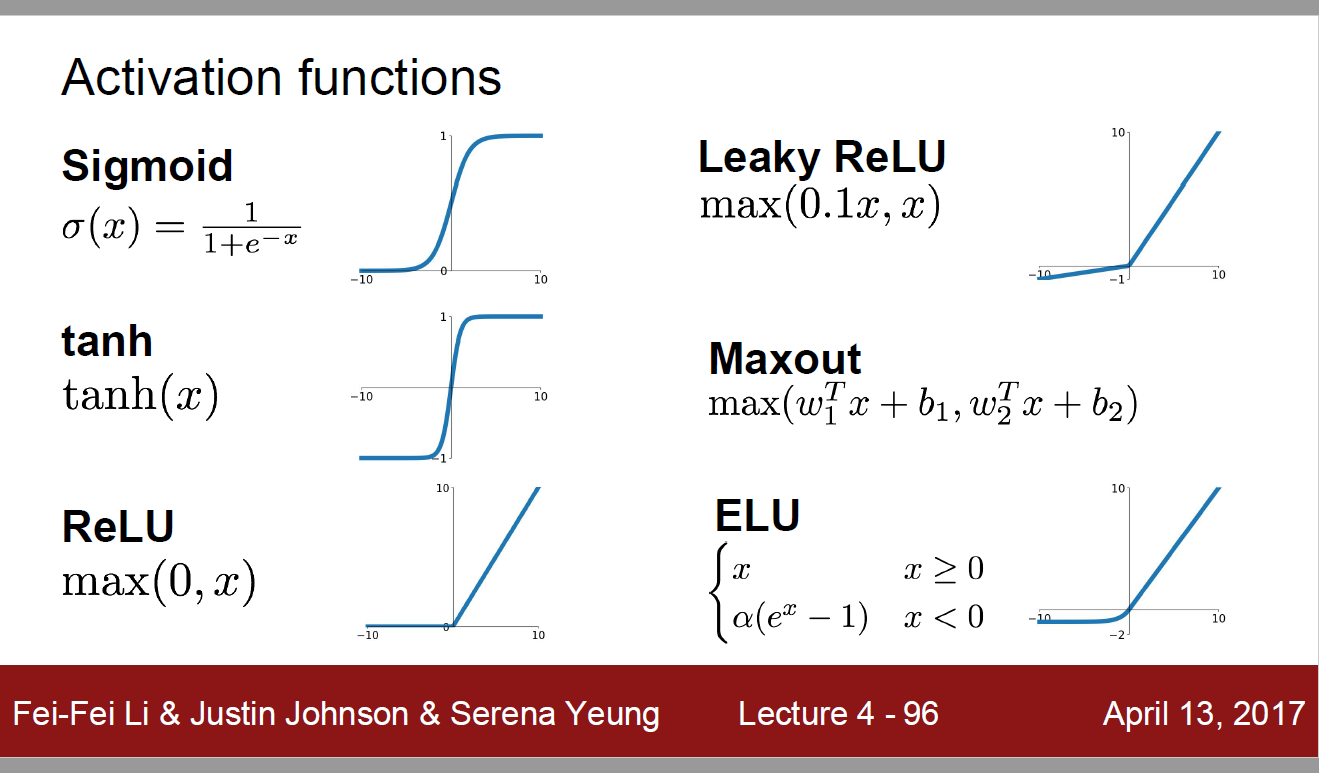sigmoid函数（也叫逻辑斯谛函数）： 　引用wiki百科的定义：　　A logistic function or logistic curve is a common “S” shape (sigmoid curve).　　其实逻辑斯谛函数也就是经常说的sigmoid函数，它的几何形状也就是一条sigmoid曲线。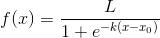其反向传播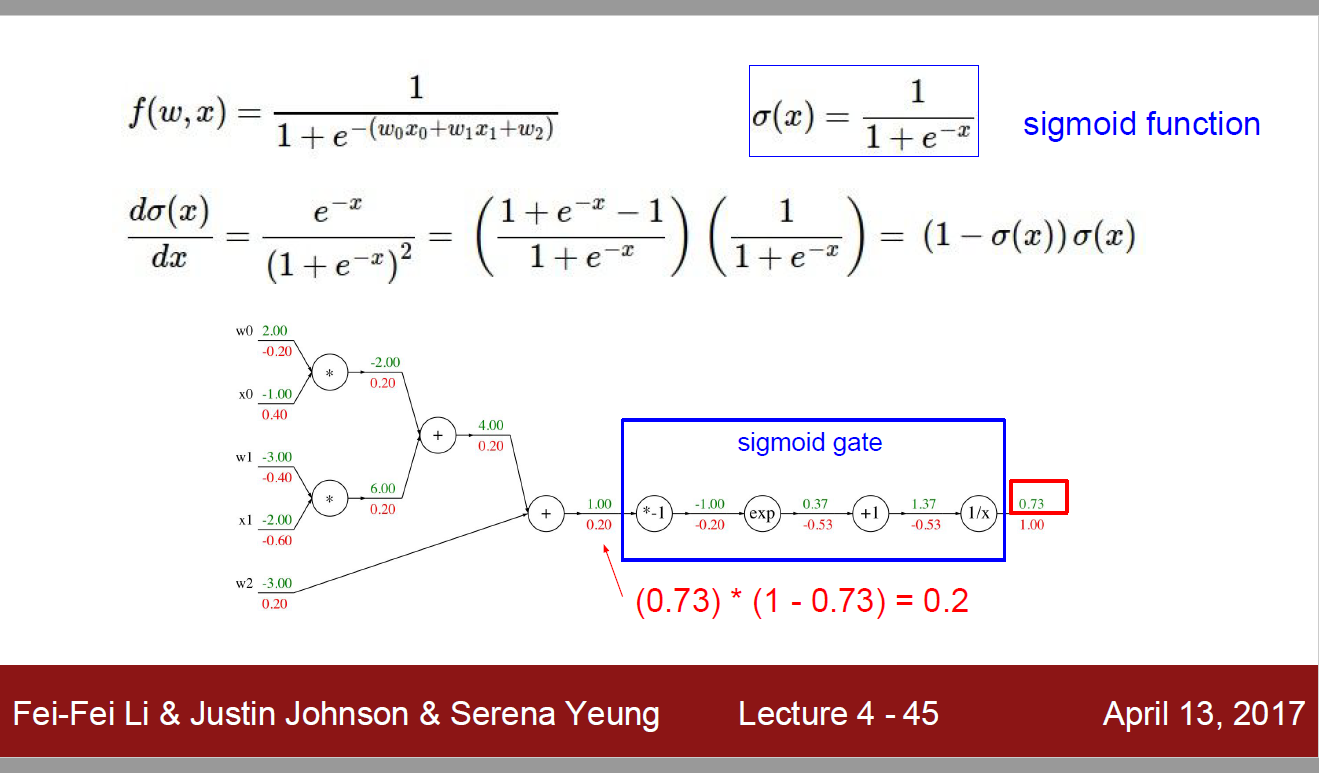同样，我们贴一下wiki百科对softmax函数的定义：softmax is a generalization of logistic function that “squashes”(maps) a K-dimensional vector z of arbitrary real values to a K-dimensional vector σ(z) of real values in the range (0, 1) that add up to 1.　　这句话既表明了softmax函数与logistic函数的关系，也同时阐述了softmax函数的本质就是将一个K 维的任意实数向量压缩（映射）成另一个K。不作为激活函数维的实数向量，其中向量中的每个元素取值都介于（0，1）之间。　　softmax函数形式如下：总结：sigmoid将一个real value映射到（0,1）的区间（当然也可以是（-1,1）），这样可以用来做二分类。 而softmax把一个k维的real value向量（a1,a2,a3,a4….）映射成一个（b1,b2,b3,b4….）其中bi是一个0-1的常数，然后可以根据bi的大小来进行多分类的任务，如取权重最大的一维。 同样，我们贴一下wiki百科对softmax函数的定义：softmax is a generalization of logistic function that “squashes”(maps) a K-dimensional vector z of arbitrary real values to a K-dimensional vector σ(z) of real values in the range (0, 1) that add up to 1.　　这句话既表明了softmax函数与logistic函数的关系，也同时阐述了softmax函数的本质就是将一个K 维的任意实数向量压缩（映射）成另一个K维的实数向量，其中向量中的每个元素取值都介于（0，1）之间。　　softmax函数形式如下：总结：sigmoid将一个real value映射到（0,1）的区间（当然也可以是（-1,1）），这样可以用来做二分类。 而softmax把一个k维的real value向量（a1,a2,a3,a4….）映射成一个（b1,b2,b3,b4….）其中bi是一个0-1的常数，然后可以根据bi的大小来进行多分类的任务，如取权重最大的一维。
展开全文• ReLU函数, 即线性整流函数(Rectified Linear Unit), 是神经网络结构中常用的非线性激活函数. 其定义如下: ReLU(x)={0,x⩽0x,x&amp;amp;amp;amp;amp;amp;amp;amp;amp;amp;amp;gt;0 ReLU(x) = \left\{ \...
摘要
本文给出 ReLU 函数的定义, 并求解其在反向传播中的梯度
相关
配套代码, 请参考文章 :
Python和PyTorch对比实现ReLU函数及反向传播
系列文章索引 :
https://blog.csdn.net/oBrightLamp/article/details/85067981
正文
1. 定义
ReLU函数, 即线性整流函数(Rectified Linear Unit), 是神经网络结构中常用的非线性激活函数.
其定义如下:
$ReLU(x) = \left\{ \begin{array}{rr} 0, & x \leqslant 0\\ x, & x > 0 \end{array} \right.$
函数图像 :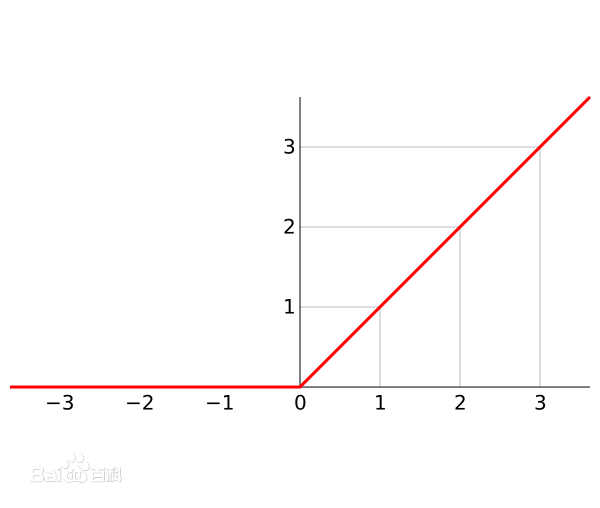2. 反向传播
ReLU 函数是逐个处理输入值的, 并不需要写成向量的形式.
但为了方便编程实现, 这里将其进行改编成向量的形式.
考虑一个输入向量 x , 经 ReLU 函数变换后得到向量 r, 往前 forward 传播得到误差值 error (标量 e ),  求 e 对 x 的梯度.
$x =( x_1,x_2,x_3,\cdots,x_k) \\ \;\\ r = ReLU(x)\\ \;\\ e=forward(r)$
求解过程 :
$\frac{d r_i}{d x_i} = \left\{ \begin{array}{rr} 0, & x_i \leqslant 0\\ 1 , & x_i > 0 \end{array} \right.\\ \;\\ m = (\frac{\partial r_1}{\partial x_1},\frac{\partial r_2}{\partial x_2},\frac{\partial r_3}{\partial x_3}, \cdots ,\frac{\partial r_k}{\partial x_k}) \\ \;\\ \nabla e_{(r)} = (\frac{\partial e}{\partial r_1},\frac{\partial e}{\partial r_2},\frac{\partial e}{\partial r_3}, \cdots ,\frac{\partial e}{\partial r_k}) \\ \;\\ \frac{\partial e}{\partial x_i} = \frac{\partial e}{\partial r_i}\frac{\partial r_i}{\partial x_i} \\ \;\\ \nabla e_{(x)} = (\frac{\partial e}{\partial x_1},\frac{\partial e}{\partial x_2},\frac{\partial e}{\partial x_3}, \cdots ,\frac{\partial e}{\partial x_k}) =\nabla e_{(r)} \odot m \;\\$
向量 $\nabla e_{(r)}$ 由上游负责计算, 是已知的, x 确定时 m 也是已知的, $\odot$ 表示元素积, 即同位元素相乘.
3. ReLU 函数的作用
为了理解 ReLU 函数的作用, 我们引入一种在电子电路中广泛应用的电子元器件 :二极管 (英语：Diode)，电子元件当中, 一种具有两个电极的装置, 只允许电流由单一方向流过, 用于整流电路，可以把交流电变换成脉动的直流电, 即整流二极管 (rectifier diode).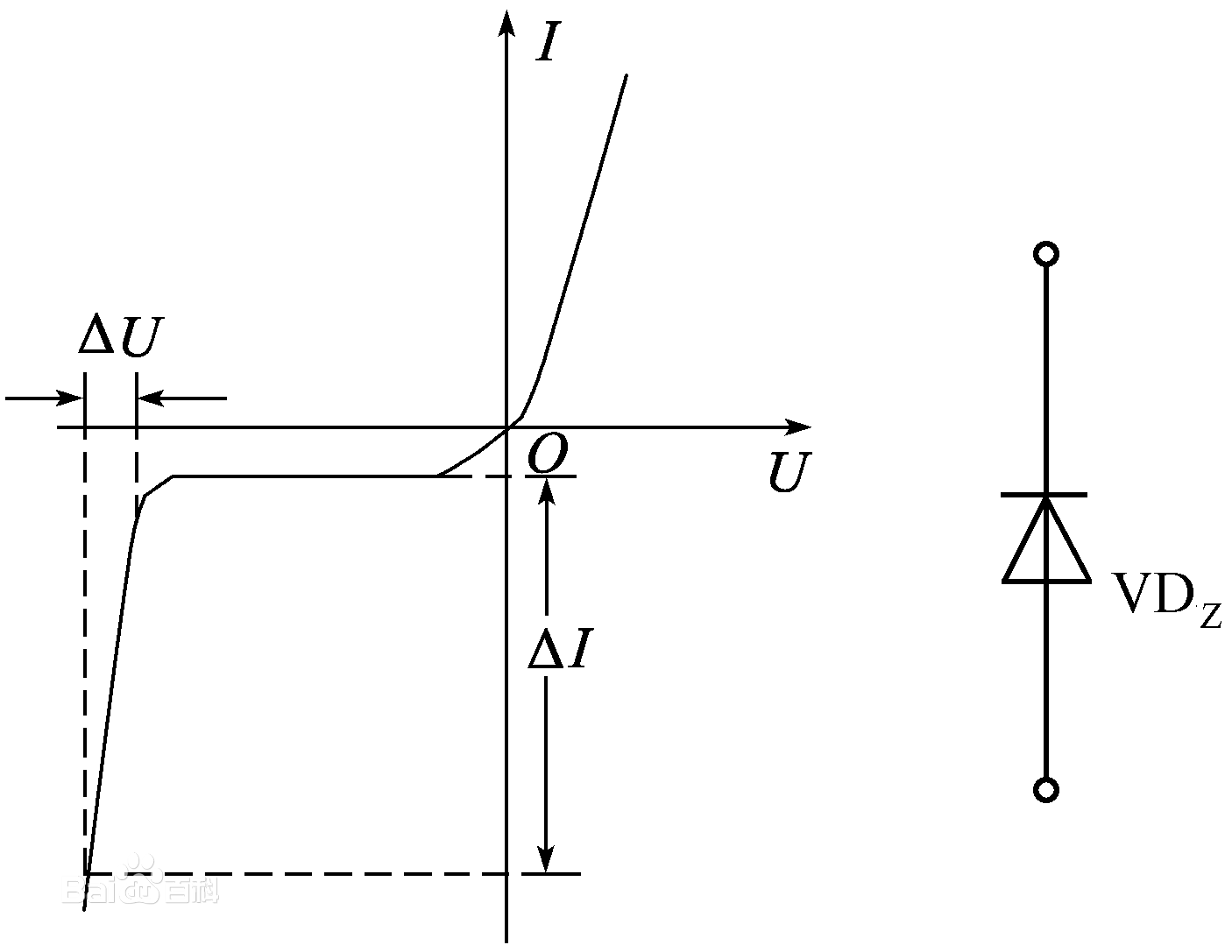上图左边是整流二极管的特性曲线, 右边是整流二极管在电路图中的表示符号.
左边$\triangle U$部分是击穿电压, 很容易烧毁二极管, 不是整流二极管的正常工作范围.
可以看到, 正常工作范围的特性曲线和 ReLU 函数的图像非常相似.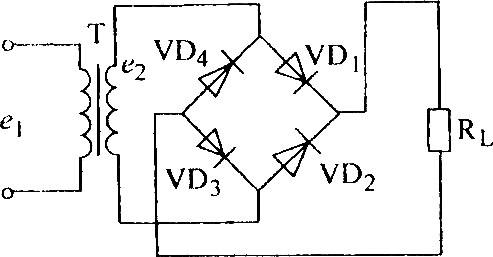上图是常见的整流电路, 利用电流只能在二极管中单向传播的特性, 通过四只二极管组合, 就可以很方便将来自 T 的交流电转换为$R_L$使用的直流电.
使用 Python 模拟整流电路 :
import numpy as np
import matplotlib.pyplot as plt

def relu(signal):
return np.maximum(0, signal)

def relu_reverse(signal):
return -np.minimum(0, signal)

x = np.linspace(-10, 10, 2000)
y = np.sin(x)

plt.axhline(color="black")
plt.axvline(color="black")
plt.plot(x, y)
plt.show()

y = relu(y) + relu_reverse(y)

plt.axhline(color="black")
plt.axvline(color="black")
plt.plot(x, y)
plt.show()

整流前 :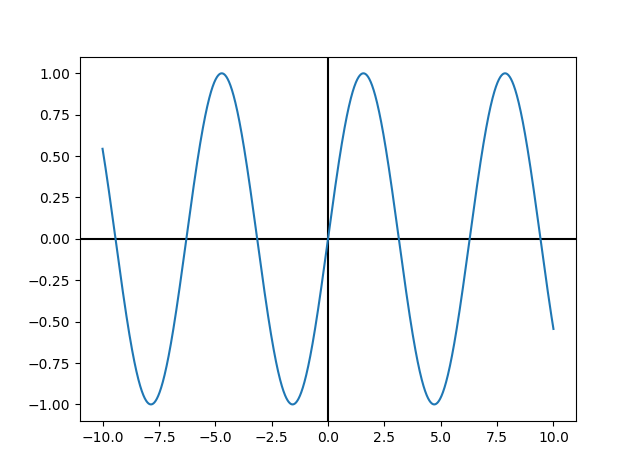整流后 :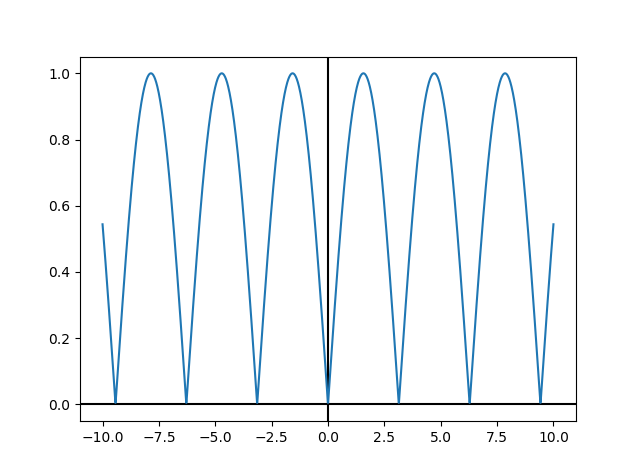可以看到, 经过整流后, 所有的反向信号都调整为正向信号了.
4. ReLU + 线性组合
在常见的神经网络结构中, ReLU 经常和线性变换一起使用.
$a_i = a_ix_i + b_i\\ \;\\ y = \sum_{k = 1}^{k} ReLU(a_i)$
import numpy as np
import matplotlib.pyplot as plt

def relu(signal):
return np.maximum(0, signal)

x = np.linspace(-10, 10, 2000)

b1 = 0.00 * np.pi
b2 = 0.25 * np.pi
b3 = 0.50 * np.pi
b4 = 0.75 * np.pi
b5 = 1.00 * np.pi
b6 = 1.25 * np.pi
b7 = 1.50 * np.pi
b8 = 1.75 * np.pi

y1 = np.sin(x + b1)
y2 = np.sin(x + b2)
y3 = np.sin(x + b3)
y4 = np.sin(x + b4)
y5 = np.sin(x + b5)
y6 = np.sin(x + b6)
y7 = np.sin(x + b7)
y8 = np.sin(x + b8)

y = relu(y1) + relu(y2) + relu(y3) + relu(y4)
y += relu(y5) + relu(y6) + relu(y7) + relu(y8)

plt.plot(x, y)
plt.axhline(color="black")
plt.axvline(color="black")
plt.show()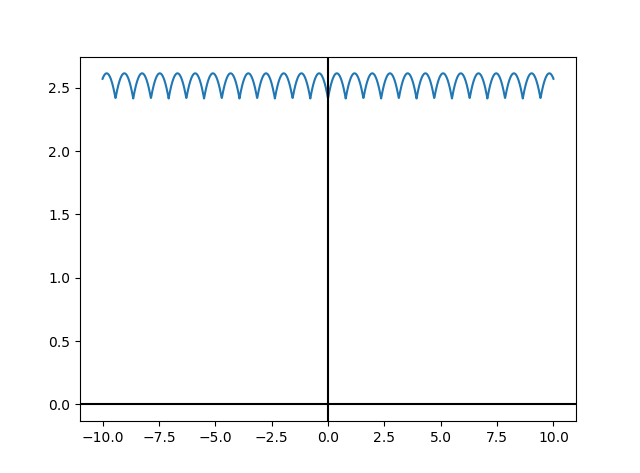只需要有限的几个 ReLU 进行组合, 就可以将交流信号调整到不错的直流信号.
ReLU 函数虽然很简单, 但和其他函数组合后却有神奇的非线性拟合能力.


展开全文• 在使用relu激活函数时，我在实现backprop时遇到了问题。我的模型有两个隐藏层，在两个隐藏层中有10个节点，在输出层中有一个节点(因此3个权重，3个偏差)。我的模型不适用于这个坏掉了的向后推功能。但是，该函数使用...
• ## RELU函数理解

千次阅读 2015-07-01 14:10:17
在CNN卷积神经网络中，习惯用RELU函数代替sigmoid, tahh等目标激活函数，这应该是因为，RELU函数在大于0的时候，...导数小的话，在训练神经网络的时候，会BP反向传播误差，导致收敛减慢；而relu函数则避免了这点，很好
• 1.relu/tanh激活函数 Q:为什么要有激活函数？ A:如果没有激活函数，那么输出只是输入的线性组合，意义不大 比如:有输入x1,x2…xn,如果没有激活函数，最终的输出可以写成表达式 z=a1×x1+a2×x2+..an×xnz=a1\times ...
• 依赖知识 ReLU 前向过程 后向过程 ...本文介绍常见的激活函数的前向过程及反向传播过程 依赖知识 a) 熟悉全连接层、损失函数反向传播 b) 熟悉ReLU、LeakyReLU、PReLU、ELU、SELU ReLU ​...
• https://blog.csdn.net/oBrightLamp/article/details/84326091
• 1 饱和神经元会杀死梯度,离中心越远的x处的导数接近于0,停止反向传播的学习过程 2 容易饱和,一直是正数0到1之间的数,以0.5为中心,求权重的梯度时,梯度总是正或者总是负 3 指数计算耗时 4层以内网络可以用...
• ReLU函数（ReLU Function） 本节通过实例介绍ReLU函数。...DeepReLU函数利用反向传播算法对给定的深度神经网络进行训练。 The function DeepReLU trains the given deepneural network using the ba...
• ## sigmoid与ReLU函数

千次阅读 2017-02-21 17:52:56
单极性Sigmoid函数 单极性Sigmoid函数，即f(x)=11+e−x,f(x)′=f(x)[1−f(x)]f(x)=\frac{1}{1+e^{-x}},f(x)'=f(x)[1-f(x)],该传输...sigmoid函数提供概率解释，另外，sigmoid函数是可微的，所以用于反向传播算法（B
• 1.神经网络激活函数介绍 1.Relu函数的导数计算
• relu_layer实际采用leaky_relu作为激活函数，普通relu优缺点如下： ReLU 的优点： Krizhevsky et al. 发现使用 ReLU 得到的SGD的收敛速度会比 sigmoid/tanh 快很多(看右图)。有人说这是因为它是linear，...
• 激活函数是神经网络中必不可少的一个环节，它将输入和输出通过函数映射关联起来，激活函数是非线性函数，例如Relu函数、Sigmoid函数、Tanh函数。 提示：以下是本篇文章正文内容，有些许内容及图片借鉴于《深度学习...神经网络 机器学习
• 正向传播x>0，反向传播时，则会将上游的值原封不动的传递给下游 正向传播x<... """Relu函数反向传播时，x>0则会将上游的值原封不动的传递给下游（dx = dout） x<0则会将信号停在这里（dout=...
• ## ReLU激活函数

千次阅读 2018-03-15 10:29:42
最初期的线性激活函数(y=x)和阶跃激活函数(-1/1,0/1)就不说了Sigmod和Tanh: ...缺点：在反向传播中梯度值会逐渐减小，经过多层的传递后会呈指数级下降，导致参数更新非常缓慢，无法起到训练作用。无法适应深层的...tensorflow
• 在深度学习笔记（2）——卷积神经网络（Convolutional Neural Network）中我们介绍了CNN网络的前向传播，这一篇我们介绍CNN的反向传播，讲到反向传播的时候实质就是一大堆求梯度的数学公式，这些公式其实已经在深度...
• 1 ReLU激活函数提出的背景 1）降低计算量。 早期使用的sigmoid函数，...对于深层网络，sigmoid函数反向传播时，很容易就会出现梯度消失的情况（在sigmoid接近饱和区时，变换太缓慢，导数趋于0，这种情况会造成信息...深度学习
• 目录 前言 计算图 计算图求解示例 计算图的优点 反向传播 ...计算图的反向传播 ...激活函数ReLU反向传播 激活函数Sigmoid的反向传播 Affine/softmax激活函数反向传播 Affine层 softmax-wi...深度学习 神经网络 梯度
• 第二，对于深层网络，sigmoid函数反向传播时，很容易就会出现 梯度消失 的情况（在sigmoid接近饱和区时，变换太缓慢，导数趋于0，这种情况会造成信息丢失），从而无法完成深层网络的训练。 第三，ReLu会使一部分神经...神经网络 自然语言处理 深度学习
• 为什么使用relu? 1.使用sigmoid计算量会特别大 2.sigmoid在反向传播算法时候,随着深度加深有可能会出现梯度爆炸
• 一、激活方法(Activation Functions)给定一个或者一组输入的节点的输出1.ReLU2. Sigmoid/Logistic3.Binary4.Tanh5.Softplus6.softmax7.Maxout8....二、反向传播(Backpropagation)1.这是一种用于人造神经网络的方法，深度学习 神经网络
• （1）反向传播时，可以避免梯度消失。 （2）Relu会使一部分神经元的输出为0，这样就造成了网络的稀疏性，并且减少了参数的相互依存关系，缓解了过拟合问题的发生（以及一些人的生物解释balabala）。 （3）**相对于...
• 神经元模型：用数学公式表示为：，f 为激活函数。神经网络是以神经元为基本单元构成的。 激活函数：引入非线性激活因素...① 激活函数 relu: 在 Tensorflow 中，用 tf.nn.relu()表示 ② 激活函数 sigmoid：...神经网络
• 以下代码通过使用ReLU作为激活函数构建反向传播神经网络实现了XOR # -*- coding: utf-8 -*- import numpy as np import matplotlib.pyplot as plt # N is batch size(sample size); D_in is input dimension; # H...神经网络 机器学习
• 激活函数导数1.1 Sigmoid函数导数1.2 ReLU 函数导数1.3 LeakyReLU函数导数1.4 Tanh 函数梯度2.链式法则3.反向传播算法实战 反向传播算法 1.激活函数导数 1.1 Sigmoid函数导数 Sigmoid函数表达式：σ(x)=11+e−x\...深度学习 神经网络 tensorflow 机器学习...